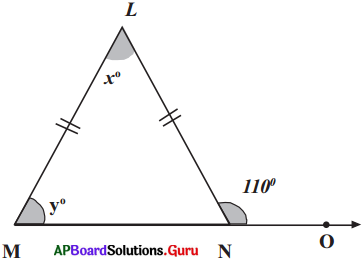SCERT AP 7th Class Maths Solutions Pdf Chapter 5 Triangles InText Questions and Answers.

## AP State Syllabus 7th Class Maths Solutions 5th Lesson Triangles InText Questions

Check Your Progress [Page No. 92]

Question 1.
Classify the following triangles according to the length of their sides: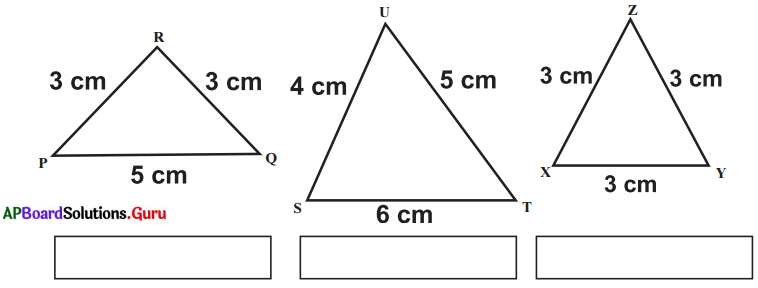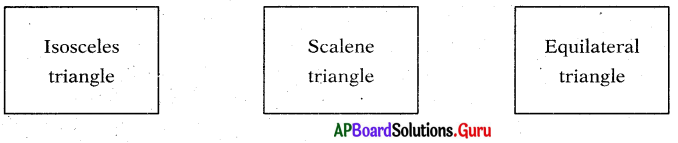[Page No. 93]

Question 2.
Classify the following triangles according to the measure of their angles.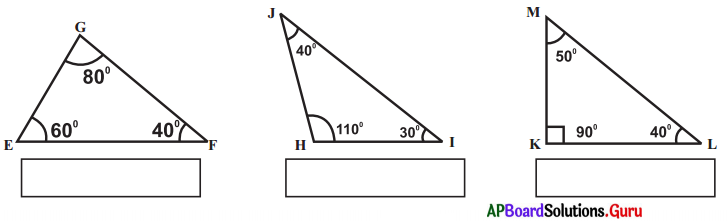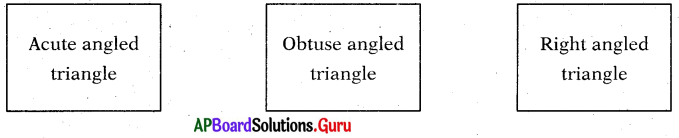Let’s Think [Page No. 97]

Question 1.
Can you find a triangle in which each angle is less than 60°?
Sum of angles in a triangle is 180°.
If each angle is less than 60°, then the sum of angles of a triangle is < 180°. So, triangle cannot form. So, we cannot find a triangle in which each angle is less than 60°.Check Your Progress [Page No. 99]

Question 1.
Find the value of ‘x’ in the given figure.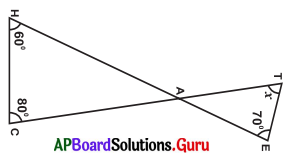In the given figure,
∠HAC = ∠TAE = a (∵ Vertically opposite angles)
In ∆AHC,
we know, ∠H + ∠C + ∠HAC 180°
⇒ 60° + 80° + a = 180°
⇒ 140° + a – 140° = 180°- 140° … a = 40°.
∴ ∠HAC = ∠TAE = a = 40°

In ∆ATE, we know,
∠T + ∠TAE + ∠E = 180°
⇒ x + a + 70° = 180°
⇒ x + 40° + 70° = 180°
⇒ x + 110°- 110° = 180°- 110°
∴ x = 70°

Let’s Do Activity [Page No. 101]

Question 1.
Measure the length of the sides of ∆ABC and fill the following table.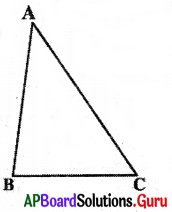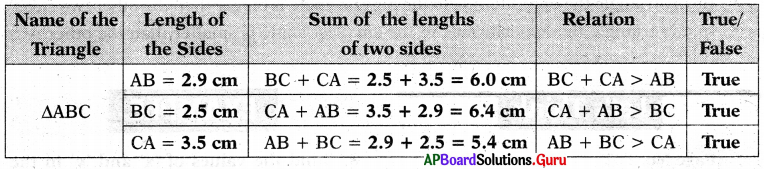From the above table, we can conclude that the sum of the lengths of any two sides of a triangle is greater than the length of the third side.

Question 2.
Take the same measurements of the previous activity and note the results as given below.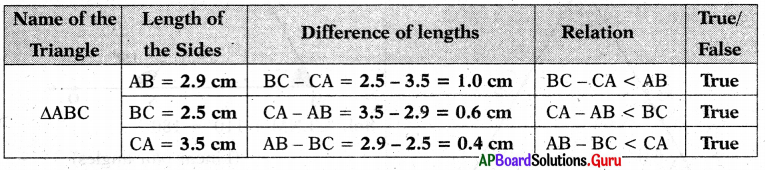From the above table we can conclude that the difference of the lengths of any two sides of a triangle is less than the length of the third side.

Question 3.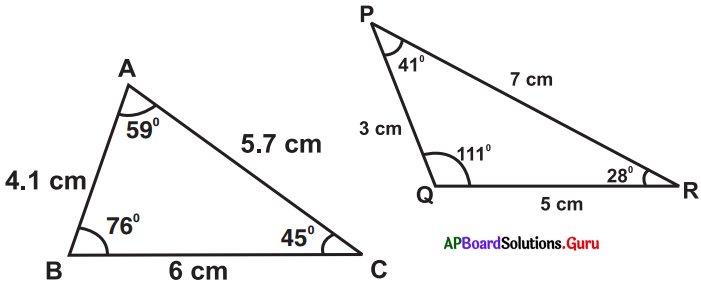Fill the following table as per measurements given in the above triangles: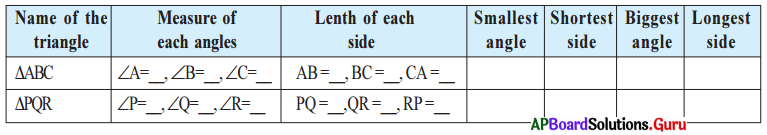What do you observe from the last four columns in above table ?

• In any triangle the opposite side to the biggest angle is bigger than the other two sides.
• In any triangle the opposite side to the smallest angle is smaller than the other two sides.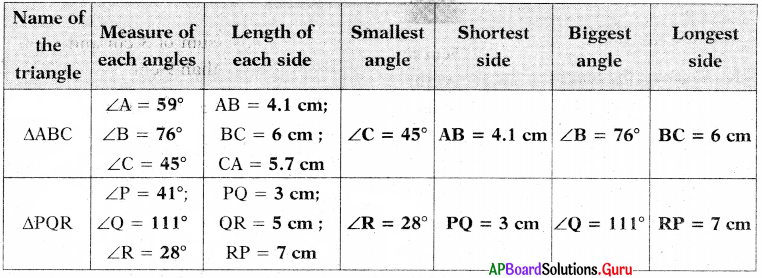Let’s Think [Page No. 102]

Question 1.
What are the measurements of angles of an equilateral triangle?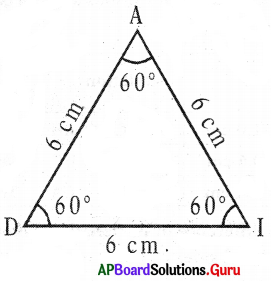AD = DI – AI = 6 cm (All angles are equal)
∠A = ∠D = ∠I = 60° (All angles are equal)
So, in an equilateral triangle,

All Sides are equal in length. Each angle is equal to 60°.Puzzle Time [Page No. 104]

Question 1.
Find the interior angles of all triangles by using given dues.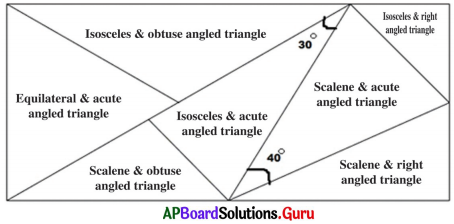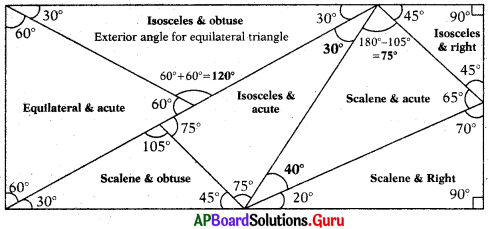Hints:
Each angle in an equilateral triangle is 60°.
Straight angle = 180°
In an isosceles triangle, two angles are equal.
In a scalene triangle all angles are unequal.
Right angle = 90°.
Obtuse angle > 90°.

Examples:

Question 1.
In a triangle, two angles are 43° and 57°. Find the third angle.
Given two angles of a triangle are 43° and 57°.
Sum of these two angles = 43° + 57° = 100°
In a triangle the sum of the interior angles is 180°.
∴ Third angle = 180° – 100° = 80°

Question 2.
Find the value of ‘x’ in the following triangles :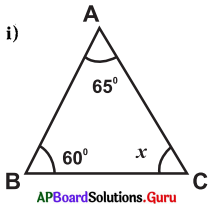From the figure
∠A + ∠B + ∠C = 180° (v Sum of three angles in triangle is 180°)
⇒ 65° + 60° + x = 180°
⇒ 125° + x = 180°
∴ x =180°- 125° = 55°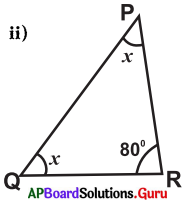From the figure
∠P + ∠Q + ∠R = 180°
x + x + 80° = 180°
⇒ 2x + 80° = 180°
⇒ 2x = 180°-80°
⇒ 2x = 100°
⇒ x = $$\frac{100^{\circ}}{2}$$ = 50°
∴ x = 50°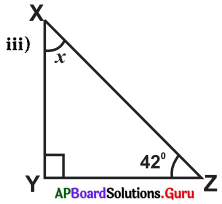From the figure
∠X + ∠Y + ∠Z – 180°
⇒ x + 90° + 42° = 180°
⇒ x + 132° = 180°
⇒ x = 180°- 132°
∴ x = 48°

Question 3.
Find the values of x and y in the given triangle.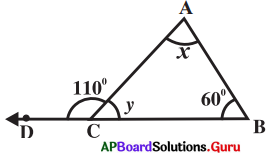In the ∆ABC
∠ACD + ∠ACB = 180° (linear pair of angles)
110° + y = 180°
⇒ y = 180°- 110°
∴ y = 70° ……………….(i)

∠BAC + ∠ACB + ∠CBA = 180°
⇒ x + y + 60° = 180°
⇒ x + 70° + 60° = 180° (From (i))
⇒ x + 130° = 180°
∴ x = 50°Question 4.
In a right angled triangle one acute angle is 44°, then find the other acute angle.
We know that, sum of the two acute angles in a right angled triangle is 90°.
Given that, in the right angled tri-angle one of the acute angles = 44°
Other acute angle in right angled triangle = 90° – 44° = 46°

Question 5.
The angles of a triangle are (x + 10)°, (x – 20)° and (x + 40)°. Find the value of x and the measure of the angles.
Given that the angles of the triangle are
(x + 10)°, (x – 20)° and (x + 40)°
(x + 10)° + (x – 20)° + (x + 40)° = 180°
⇒ x + 10° + x – 20° + x + 40° = 180°
⇒ 3x + 30° = 180°
⇒ 3x – 180° – 30°
⇒ 3x = 150°
⇒ x = $$\frac{150^{\circ}}{3}$$ = 50°

The angles are,
x + 10° = 50° + 10° = 60°
x – 20° = 50° – 20° = 30°
x + 40° = 50° + 40° = 90°
∴ Measure of the angles are 60°, 30° and 90°.

Question 6.
In the ∆ABC, exterior angle at ∠C = 105° and ∠A=65°. Find the other interior opposite angle.
Given that, exterior angle at ∠C = 105°.
One of the interior opposite angle ∠A = 65°.

The other interior opposite angle is ∠B .
∠A + ∠B =105°(∵ Exterior angle property of a triangle)
65° + ∠B = 105°
⇒ ∠B = 105° – 65° = 40°

Question 7.
Find the exterior angle of the given triangle.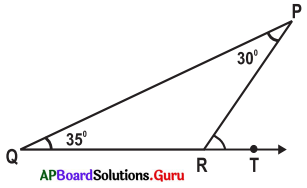From the figure, ∠P = 30°, ∠Q = 35°
Exterior angle at R = ∠P + ∠Q (∵ Exterior angle property of a triangle)
= 30° + 35° = 65°Question 8.
Find the values of ‘x’ and ‘y’ in the following figure.